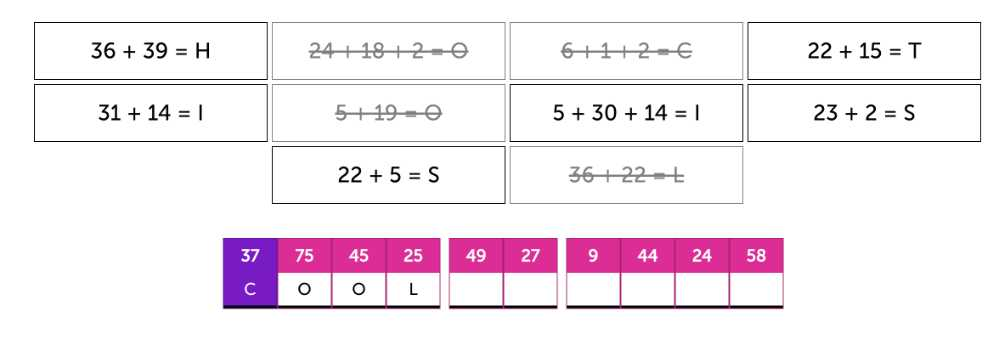# Math puzzles

By using the puzzle types in creative ways you can even turn them into all sorts of math puzzles

## 1: Creating a math puzzle within a crossword

The crossword puzzles have no restrictions in terms of what kind of characters can be used and so you can use numbers and even math symbols within your puzzles.

You can create all sort of sums, equations and other mathematical challenges to challenge the player in a fresh puzzling way :)

Crossword Puzzle maker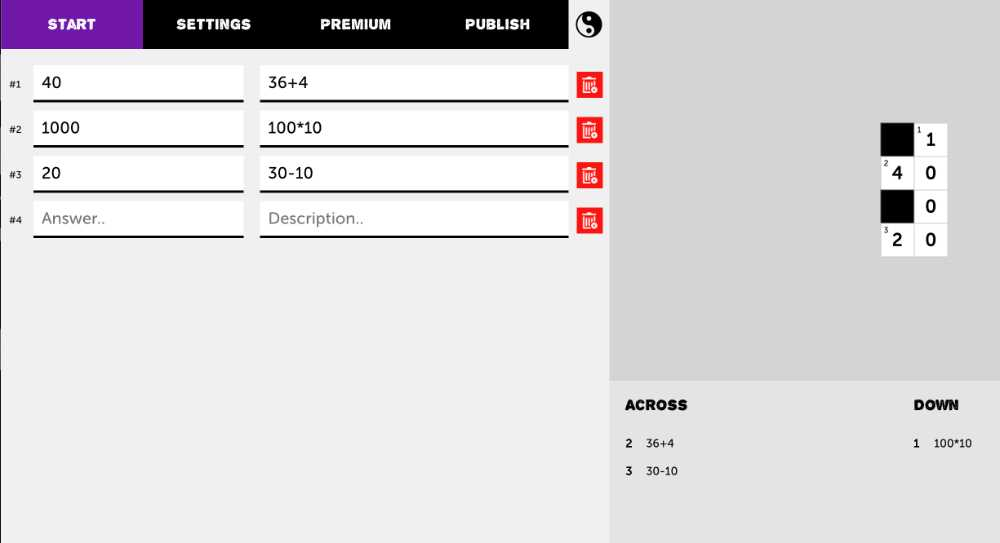## 2: Math puzzles through matching pairs

You can create cool drag and drop math puzzles via the 'Matching Pairs' puzzle type.

Enter the answer as the first field in the card pair and the question/equation in the other field. This will allow players to search for the right combination and solve the math puzzles!

Matching Pairs maker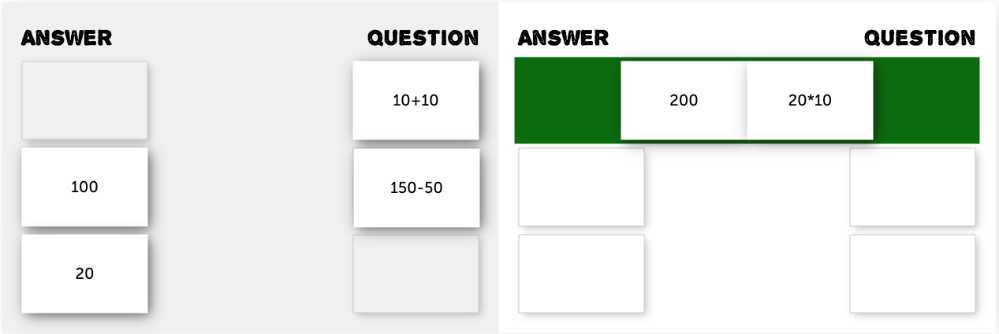## 3: Next level Memory game with math

Just like with the matching pairs, card pairs allow you to create all kinds of creative exercises, including math puzzles.

Memory is quite a bit harder for the players though, since the cards only have certain amount of 'Flip time' and then switch back. This will challenge the players to remember the question/equation and match it with the answer card.

Nonetheless, I would say this can be really fun to try out and gamify any math subject

Memory game maker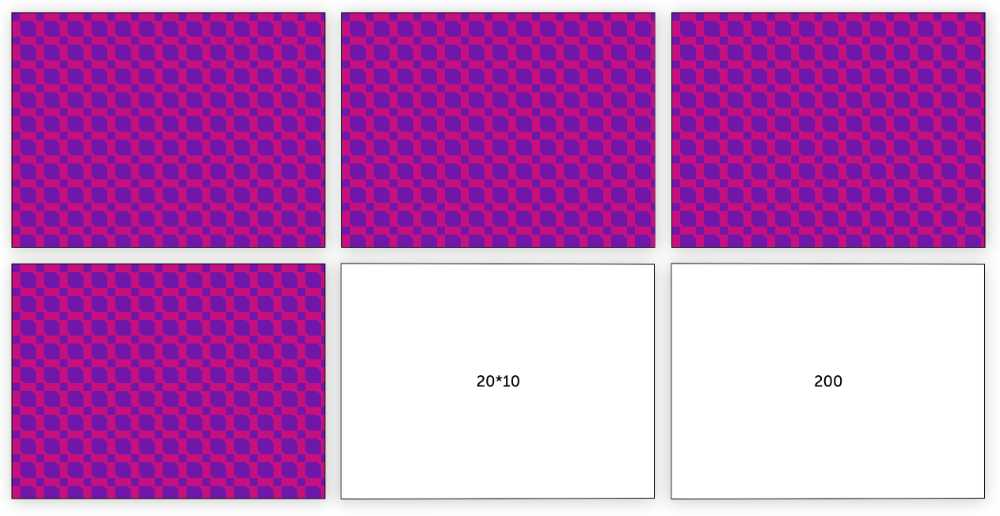## 4: Fill in the gap math puzzles

The most free format and therefore it can allow any sort of puzzle creation, including math puzzles.

You can enter any sentence and as long as you separate each symbol and number with a space, they can separately be 'gapified'. This way each question sheet can be turned into an online puzzle exercise.

Answers can vary from adding the correct numbers to finding the correct symbols to whatever you can think of in terms of math challenges.

Create your 'Fill in the gap' here:

Fill in the gap maker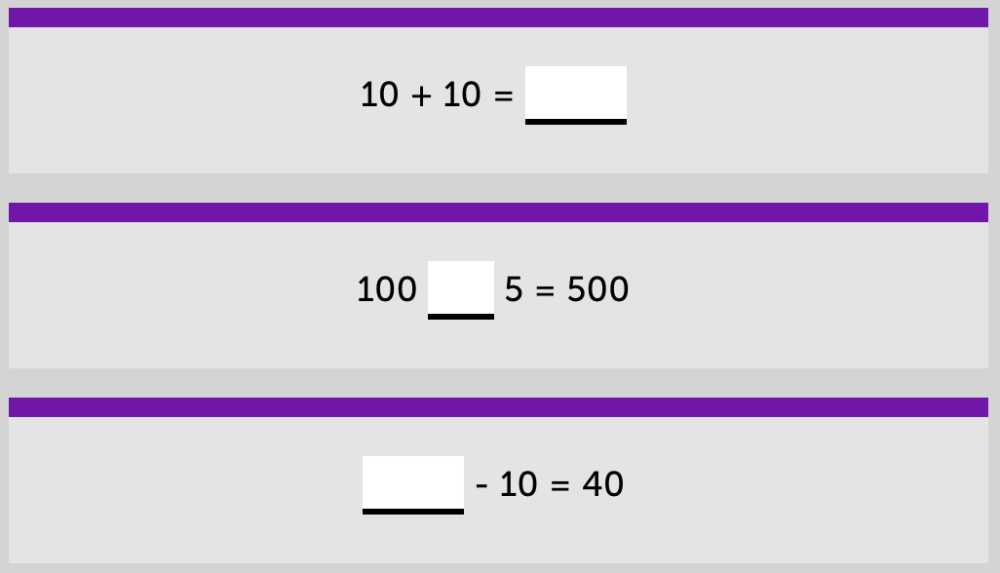## 5: Calculation puzzle

The newest puzzle addition which allows for mathematical challenges! It is the most direct embodiment of setting the player up with a math puzzle since it is only that.

You only have to think of a hidden solution, tweak the difficulty and the calculation puzzle algorithm does the rest! Due to the many settings, you can easily get a customized mathematical exercise that is just right for your students.